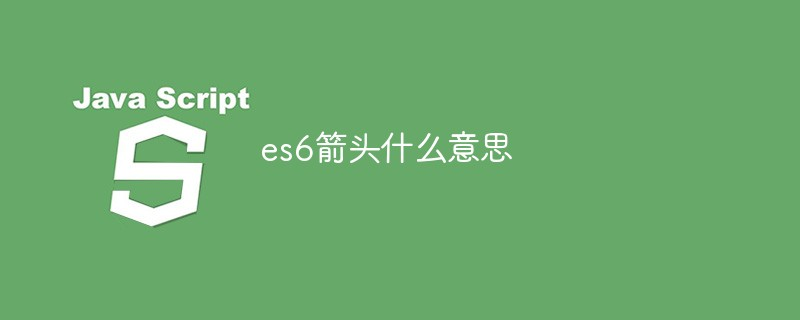# es6箭头什么意思ES6标准新增了一种新的函数：Arrow Function（箭头函数）。

## 基础语法

```var fn1 = function(a, b) {
return a + b
}

function fn2(a, b) {
return a + b
}```

```var fn1 = (a, b) => {
return a + b
}

(a, b) => {
return a + b
}```

```// 无参
var fn1 = function() {}
var fn1 = () => {}

// 单个参数
var fn2 = function(a) {}
var fn2 = a => {}

// 多个参数
var fn3 = function(a, b) {}
var fn3 = (a, b) => {}

// 可变参数
var fn4 = function(a, b, ...args) {}
var fn4 = (a, b, ...args) => {}```

```() => return 'hello'
(a, b) => a + b```
```(a) => {
a = a + 1
return a
}```

```x => {key: x} // 报错
x => ({key: x}) // 正确```JavaScript中的每一个Function对象都有一个apply()方法和一个call()方法

apply调用一个对象的一个方法，用另一个对象替换当前对象。例如：B.apply(A, arguments)；即A对象调用B对象的方法。func.apply(thisArg, [argsArray])
call调用一个对象的一个方法，用另一个对象替换当前对象。例如：B.call(A, args1,args2)；即A对象调用B对象的方法。func.call(thisArg, arg1, arg2, ...)## 总结

• 类似于匿名函数，在某些情况下使用，可减少代码量

• 代码简洁，this提前定义

• 代码太过简洁，导致不好阅读

• this提前定义，导致无法使用js进行一些在ES5里面看起来非常正常的操作（若使用箭头函数，在监听点击事件的回调函数中，就无法获取到当前点击的元素咯，详见《正确使用箭头函数——什么时候不该用ES6箭头函数》）

• 总的来说，箭头函数只是一种函数的简写，有其利弊，可用可不用，看大家心情，当然也得用的正确

【推荐学习：javascript高级教程• 相关标签：JavaScript es6 箭头函数
• 相关文章

相关视频